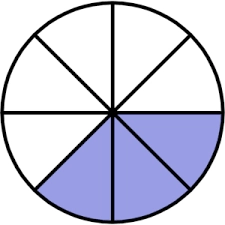# Multiply 14

Multiply 36/12 and 33/12. Write the answer as an improper fraction in lowest terms.

x =  8 14 = 33/4

### Step-by-step explanation:Did you find an error or inaccuracy? Feel free to write us. Thank you!

Tips for related online calculators
Need help calculating sum, simplifying, or multiplying fractions? Try our fraction calculator.
Need help with mixed numbers? Try our mixed-number calculator.